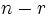Probability distribution of number of fixed points of permutations

Definition

Let$n$ be a natural number. Consider the uniform distribution on the symmetric group of degree$n$. The probability distribution of number of fixed points is the probability distribution on the set$\{ 0,1,2,\dots,n \}$ where the probability associated with$r$ is the probability that a given permutation has$r$ fixed points.

The probability distribution is given explicitly by:$\! \operatorname{Pr}(n,r) = \frac{D_{n-r}}{r!(n - r)!}$

where$D_{n-r}$ is the derangement number, i.e., the number of permutations on a set of size$n - r$ with no fixed point.

The mean (expected value) of this distribution is one. Further information: expected number of fixed points of permutation equals one

Particular cases

The first table lists number of permutations. The probabilities are obtained by dividing these numbers by$n!$. The column headers are for number of fixed points.$n$$n!$ 0 1 2 3 4 5 6 7 8
1 1 0 1
2 2 1 0 1
3 6 2 3 0 1
4 24 9 8 6 0 1
5 120 44 45 20 10 0 1
6 720 265 264 135 40 15 0 1
7 5040 1854 1855 924 315 70 21 0 1
8 40320 14833 14832 7420 2464 630 112 28 0 1

The second table gives the probabilities:$n$ 0 1 2 3 4 5 6 7 8
1 0 1
2 1/2 0 1/2
3 1/3 1/2 0 1/6
4 3/8 1/3 1/4 0 1/24
5 11/30 3/8 1/6 1/12 0 1/120
6 53/144 11/30 3/16 1/18 1/48 0 1/720
7 103/280 53/144 11/60 1/16 1/72 1/240 0 1/5040
8 2119/5760 103/280 53/288 11/180 1/64 1/360 1/1440 0 1/40320

Computer package implementation

Mathematica implementation

We need the Combinatorica package, that can be loaded using:

<< Combinatorica`

To determine the probability that a permutation on$n$ letters has$r$ fixed points, do:

Binomial[n, r] * NumberOfDerangements[n - r]/Factorial[n]

To output the whole list for a given$n$, do:

(Binomial[n, #] * NumberOfDerangements[n - #]/Factorial[n]) & /@ Range[0, n]

This can be further mapped for small values of$n$; for instance:

f[n_] := (Binomial[n, #] * NumberOfDerangements[n - #]/Factorial[n])  & /@ Range[0, n]

f /@ Range